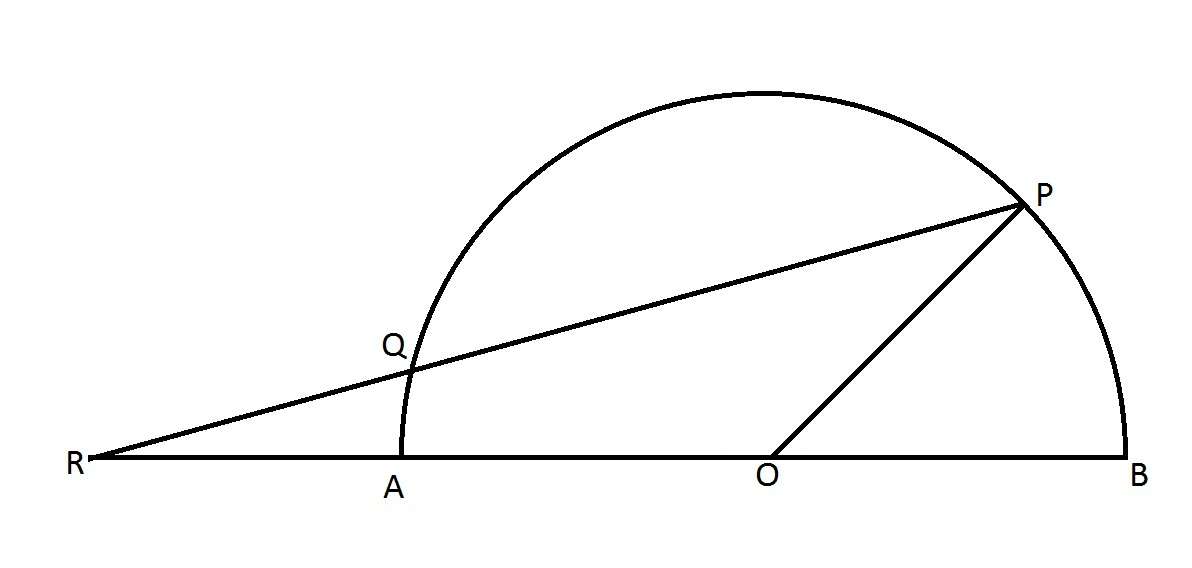# Find The PRO Angle

Geometry Level 2O is the center of the semicircle. If RQ = BO and the measure of $\angle$BOP is 60 (in degrees), then find the measure of $\angle$PRO (in degrees).

×

Problem Loading...

Note Loading...

Set Loading...